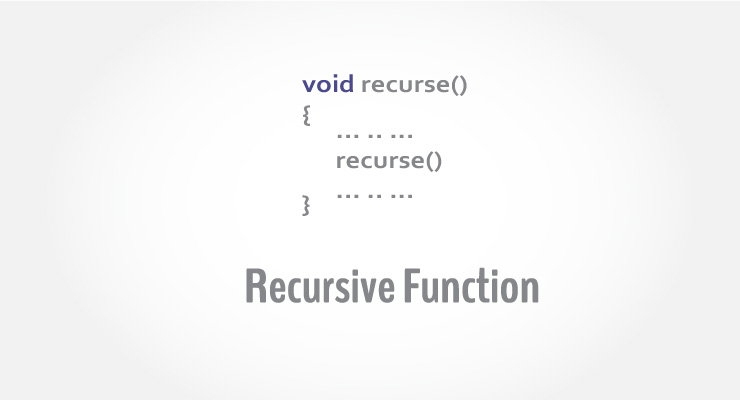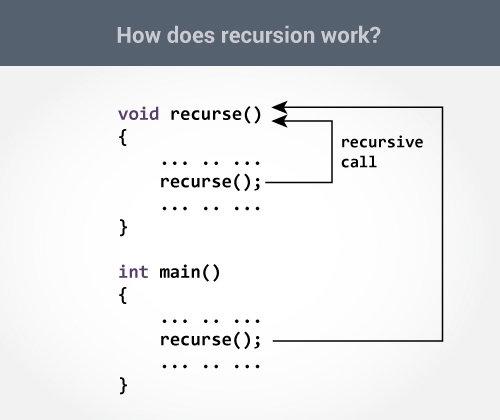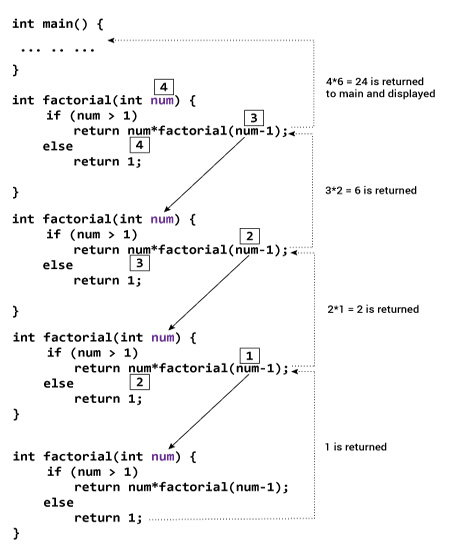# C++ Recursion

In this article, you will learn to create a recursive function; a function that calls itself.A function that calls itself is known as recursive function. And, this technique is known as recursion.

## How recursion works in C++?

```void recurse()
{
... .. ...
recurse();
... .. ...
}

int main()
{
... .. ...
recurse();
... .. ...
}
```

The figure below shows how recursion works by calling itself over and over again.The recursion continues until some condition is met.

To prevent infinite recursion, if...else statement (or similar approach) can be used where one branch makes the recursive call and other doesn't.

## Example 1: Factorial of a Number Using Recursion

``````// Factorial of n = 1*2*3*...*n

#include <iostream>
using namespace std;

int factorial(int);

int main()
{
int n;
cout<<"Enter a number to find factorial: ";
cin >> n;
cout << "Factorial of " << n <<" = " << factorial(n);
return 0;
}

int factorial(int n)
{
if (n > 1)
{
return n*factorial(n-1);
}
else
{
return 1;
}
}``````

Output

```Enter a number to find factorial: 4
Factorial of 4 = 24```

### Explanation: How this example works?Suppose the user entered 4, which is passed to the `factorial()` function.

1. In the first `factorial()` function, test expression inside if statement is true. The `return num*factorial(num-1);` statement is executed, which calls the second `factorial()` function and argument passed is `num-1`which is 3.

2. In the second `factorial()` function, test expression inside if statement is true. The `return num*factorial(num-1);` statement is executed, which calls the third `factorial()` function and argument passed is `num-1` which is 2.

3. In the third `factorial()` function, test expression inside if statement is true. The `return num*factorial(num-1);` statement is executed, which calls the fourth `factorial()` function and argument passed is `num-1` which is 1.

4. In the fourth `factorial()` function, test expression inside if statement is false. The `return 1;` statement is executed, which returns 1 to third `factorial()` function.

5. The third `factorial()` function returns 2 to the second `factorial()` function.

6. The second `factorial()` function returns 6 to the first `factorial()` function.

7. Finally, the first `factorial()` function returns 24 to the `main()` function, which is displayed on the screen.